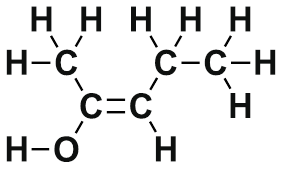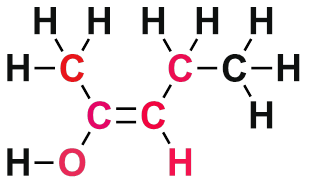# 1.8: sp² Hybrid Orbitals and the Structure of Ethylene

$$\newcommand{\vecs}{\overset { \rightharpoonup} {\mathbf{#1}} }$$ $$\newcommand{\vecd}{\overset{-\!-\!\rightharpoonup}{\vphantom{a}\smash {#1}}}$$$$\newcommand{\id}{\mathrm{id}}$$ $$\newcommand{\Span}{\mathrm{span}}$$ $$\newcommand{\kernel}{\mathrm{null}\,}$$ $$\newcommand{\range}{\mathrm{range}\,}$$ $$\newcommand{\RealPart}{\mathrm{Re}}$$ $$\newcommand{\ImaginaryPart}{\mathrm{Im}}$$ $$\newcommand{\Argument}{\mathrm{Arg}}$$ $$\newcommand{\norm}{\| #1 \|}$$ $$\newcommand{\inner}{\langle #1, #2 \rangle}$$ $$\newcommand{\Span}{\mathrm{span}}$$ $$\newcommand{\id}{\mathrm{id}}$$ $$\newcommand{\Span}{\mathrm{span}}$$ $$\newcommand{\kernel}{\mathrm{null}\,}$$ $$\newcommand{\range}{\mathrm{range}\,}$$ $$\newcommand{\RealPart}{\mathrm{Re}}$$ $$\newcommand{\ImaginaryPart}{\mathrm{Im}}$$ $$\newcommand{\Argument}{\mathrm{Arg}}$$ $$\newcommand{\norm}{\| #1 \|}$$ $$\newcommand{\inner}{\langle #1, #2 \rangle}$$ $$\newcommand{\Span}{\mathrm{span}}$$$$\newcommand{\AA}{\unicode[.8,0]{x212B}}$$

##### Objectives

After completing this section, you should be able to

1. account for the formation of carbon-carbon double bonds using the concept of sp2 hybridization.
2. describe a carbon-carbon double bond as consisting of one σ bond and one π bond.
3. explain the difference between a σ bond and a π bond in terms of the way in which p orbitals overlap.
##### Key Terms

Make certain that you can define, and use in context, the key terms below.

• pi (π) bond
• sp2 hybrid

## Bonding in EthyleneThus far valence bond theory has been able to describe the bonding in molecules containing only single bonds. However, when molecules contain double or triple bonds the model requires more details. Ethylene (commonly knows as ethene), CH2CH2, is the simplest molecule which contains a carbon carbon double bond. The Lewis structure of ethylene indicates that there are one carbon-carbon double bond and four carbon-hydrogen single bonds. Experimentally, the four carbon-hydrogen bonds in the ethylene molecule have been shown to be identical. Because each carbon is surrounded by three electron groups, VSEPR theory says the molecule should have a trigonal planar geometry. Although each carbon has fulfilled its tetravalent requirement, one bond appears different. Clearly, a different type of orbital overlap is involved.The sigma bonds formed in ethene is by the participation of a different kind of hybrid orbital. Three atomic orbitals on each carbon – the 2s, 2px and 2py – combine to form three sp2 hybrids, leaving the 2pz orbital unhybridized. Three of the four valence electrons on each carbon are distributed to the three sp2 hybrid orbitals, while the remaining electron goes into the unhybridized pz orbital. Each carbon in ethene is said to be a “sp2-hybridized carbon.” The electron configuration of the sp2 hybridized carbon shows that there are four unpaired electrons to form bonds. However, the unpaired electrons are contained in two different types of orbitals so it is to be expected that two different types of bonds will form.The shape of the sp2-hybridized orbital has be mathematically shown to to be roughly the same as that of the sp3-hybridized orbital. To minimize the repulsion between electrons, the three sp2-hybridized orbitals are arranged with a trigonal planar geometry. Each orbital lobe is pointing to the three corners of an equilateral triangle, with angles of 120° between them. Again, geometry and hybrization can be tied together. Atoms surrounded by three electron groups can be said to have a trigonal planar geometry and sp2 hybridization.The unhybridized 2pz orbital is perpendicular to the plane of the trigonal planar sp2 hybrid orbtals.In the ethylene molecule, each carbon atom is bonded to two hydrogen atoms. Thus, overlap two sp2-hybridized orbitals with the 1s orbitals of two hydrogen atoms for the C-H sigma bonds in ethylene (sp2(C)-1s(H). Consequently, consistent with the observations, the four carbon-hydrogen bonds in ethylene are identical.The C-C sigma bond in ethylene is formed by the overlap of an sp2 hybrid orbital from each carbon.The overlap of hybrid orbitals or a hybrid orbital and a 1s orbtial from hydrogen creates the sigma bond framework of the ethylene molecule. However the unhybridized pz orbital on each carbon remains.The unhybridized pz orbitals on each carbon overlap to a π bond (pi). The orbital overlap is commonly written as pz(C)-1pz(C). In general multiple bonds in molecular compound are formed by the overlap of unhybridized p orbitals. It should be noted that the carbon-carbon double bond in ethlene is made up of two different types of bond, a sigma and a pi.Overall, ethylene is said to contain five sigma bonds and one pi bond. Pi bonds tend to be weaker than sigma bonds because the side-by-side overlap the p orbitals give a less effective orbital overlap when compared to the end-to-end orbital overlap of a sigma bond. This makes the pi much easier to break which is one of the most important ideas in organic chemistry reactions as we will see in Chapter 7 and subsequent chapters.An ethylene molecule is said to be made up of five sigma bonds and one pi bond. The three sp2 hybrid orbitals on each carbon orient to create the basic trigonal planer geometry. The H-C-C bond angle in ethylene is 121.3o which is very close to the 120o predicted by VSEPR. The four C-H sigma bonds in ethylene . The carbon-carbon double bond in ethylene is both shorter (133.9 pm) and almost twice as strong (728 kJ/mol) than the carbon- carbon single bond in ethylene (154 pm & 377 kJ/mol). Each of the four carbon-hydrogen bond in ethylene are equivalent has have a length of 108.7 pm## Rigidity in Ethene

Because they are the result of side-by-side overlap (rather then end-to-end overlap like a sigma bond), pi bonds are not free to rotate. If rotation about this bond were to occur, it would involve disrupting the side-by-side overlap between the two 2pz orbitals that make up the pi bond. If free rotation were to occur the p-orbitals would have to go through a phase where they are 90° from each other, which would break the pi bond because there would be no overlap. Since the pi bond is essential to the structure of ethene it must not break, so there can be not free rotation about the carbon-carbon sigma bond. The presence of the pi bond thus ‘locks’ the six atoms of ethene into the same plane.## Exercise

1) Consider the following molecule:At each atom, what is the hybridization and the bond angle and the bond angle predicted by VSPER?

2) Please identify the types of orbitals shown in the following diagram:3)

a: Describe the orbitals which overlap to the carbon-nitrogen sigma bond and pie bond in the molecule below:b: What kind of orbital holds the nitrogen lone pair?

4) For the following molecule please indicate with atoms are being held in the same plane by the carbon-carbon double bond:## Solutions

1)

A - sp2, 120°

B - sp3, 109°

C - sp2, 120° (with the lone pairs present)

D - sp3, 109°

2)3)

a) The carbon and nitrogen atoms are both sp2 hybridized. The carbon-nitrogen double bond is composed of a sigma bond formed from two sp2 orbitals, and a pi bond formed from the side-by-side overlap of two unhybridized 2p orbitals.b) As shown in the figure above, the nitrogen lone pair electrons occupy one of the three sp2 hybrid orbitals.

4)#### Questions

Q1.8.1

Consider the following molecule:At each atom, what is the hybridization and the bond angle? At atom A draw the molecular orbital.

#### Solutions

S1.8.1

A - sp2, 120°

B - sp3, 109°

C - sp2, 120° (with the lone pairs present)

D - sp3, 109°1.8: sp² Hybrid Orbitals and the Structure of Ethylene is shared under a CC BY-SA 4.0 license and was authored, remixed, and/or curated by Steven Farmer, Dietmar Kennepohl, Krista Cunningham, Tim Soderberg, William Reusch, & William Reusch.